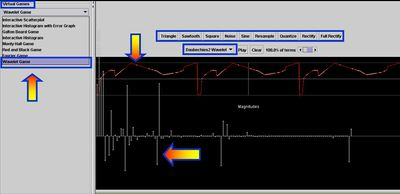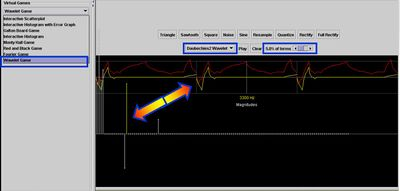# SOCR EduMaterials GamesActivitiesWavelets

## SOCR Games Activities Wavelet Game

### Summary

This activity represents a general demonstration of the effects of the Wavelet Transform on different 1D periodic signals.

### Goals

The aims of this activity are to

• demonstrate the spectral (frequency-space) representation of data (signals) using different wavelet base functions
• illustrate the effects of manually manipulating the magnitudes of the wavelet coefficients on the original signal
• justify the process of wavelet-space thresholding (wavelet shrinkage) as a tool for:
• signal denoising
• increasing the power of statistical analyses in the wavelet domain
• compact support and local effect of the wavelet base functions.

### The SOCR Wavelet Game

To start this Game, go to SOCR Games and select the Wavelet Game from the drop-down list of games in the left panel. The image below shows the interface to this game. You can select from a set of predefined periodic functions or click on the top panel and draw a new function (signal) using the mouse. Notice the wavelet representation of the signal on the bottom panel.### Experiment 1

Now we will investigate the properties of wavelet shrinkage and the effects of the position and magnitude of the wavelet coefficients. Bring the mouse over any of the wavelet coefficients in the bottom graph panel. You will see a yellow-color plot in the top panel superimposed on top of the original signal. This demonstrates the location and frequency of the corresponding wavelet base function, whose coefficient in the wavelet decomposition you have selected (by the mouse over). Now change the number of wavelet coefficients that are used to reconstruct (synthesize) the signal (choose 5% of terms, using the slider on the top). This will show you a red-color curve that is in the same space as the original signal and represents a wavelet-based signal approximation. Lastly, click on any of the large wavelet coefficients (bottom graph panel) and change its magnitude. Notice the effect of this manipulation on the approximation on the original signal (location and frequency of this effect) on the top panel. Also experiment with different wavelet bases. Notice how the number of vanishing moments effects the shape of the base functions and the corresponding wavelet encoding.

Try to pose and answer questions like: If you set to zero the wavelet coefficients in the right (or left) end of the domain, what will be the effect on the signal in time/space?